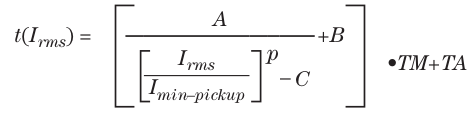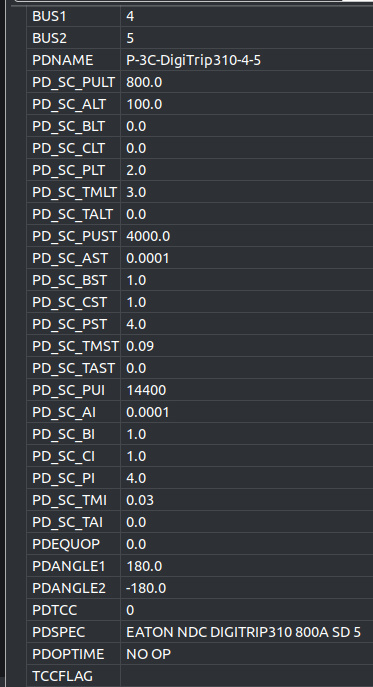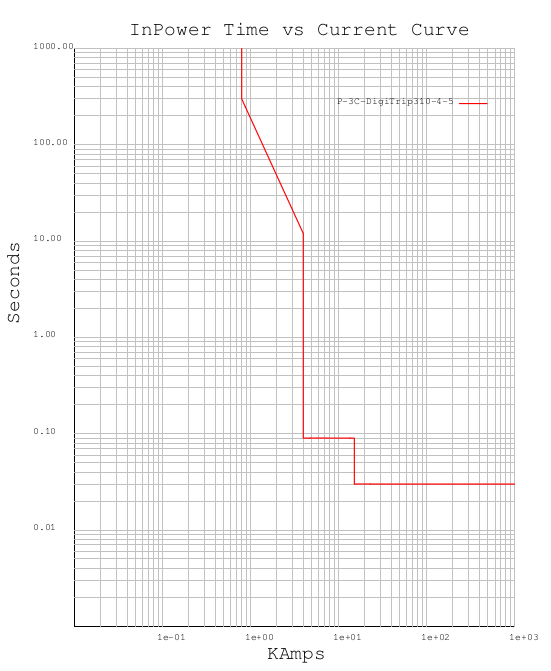# BricsCAD InPower Time-Current Characteristic Curves S&C Bulletin 680-211

One of many time current characteristic curves can be generated using the S&C document link below.

Equation 1 is used by BricsCAD InPower to generate custom time current curves (TCCs) for protective device blocks (PDEV blocks).Equation 1.

Where t is the time in seconds
A,B,C, and p are coefficients
Irms is the RMS branch current in Amps
Imin-pickup is the PDEV pick up setting in Amps
TM is the time dial setting
and TA is the time offset in seconds

For this example the BricsCAD InPower PDEV block INPOWER_PD_PHASE51_3C will be used.Figure 1. PDEV Block Attributes

This block has three TCC equations embedded in one block for any imaginable custom curve needed.

This example shows the Eaton Digitrip 310 set at 800A pickup for the long time setting (LT), 4000 Amps pickup for the short time setting (ST) and 14,000 Amps for the instantaneous pickup setting (I).

BUS1 and BUS2 are the branch the PDEV is protecting
PDANGLE1 and 2 are the directions attributes if needed
PDTCC turns on the TCC plotting for this PDEV.
PDSPEC are the notes and specifications for this PDEV
PDOPTIME is the operation time of this PDEV set by InPower
TCCFLAG is the flag set by InPower for the active TCC

The other attributes are the three curves described by equation 1 above

Shown below is the TCC plot for the S&C three curve PDEVFigure 2. TCC Plot For The Digitrip 310

Care must be taken so that the LT, ST and I curves do not overlap in an unintentional way.
Use this link as a guide to help with the coefficients.

https://www.sandc.com/globalassets/sac-electric/documents/public---documents/sales-manual-library---external-view/information-bulletin-680-211.pdf?dt=638136391682871389